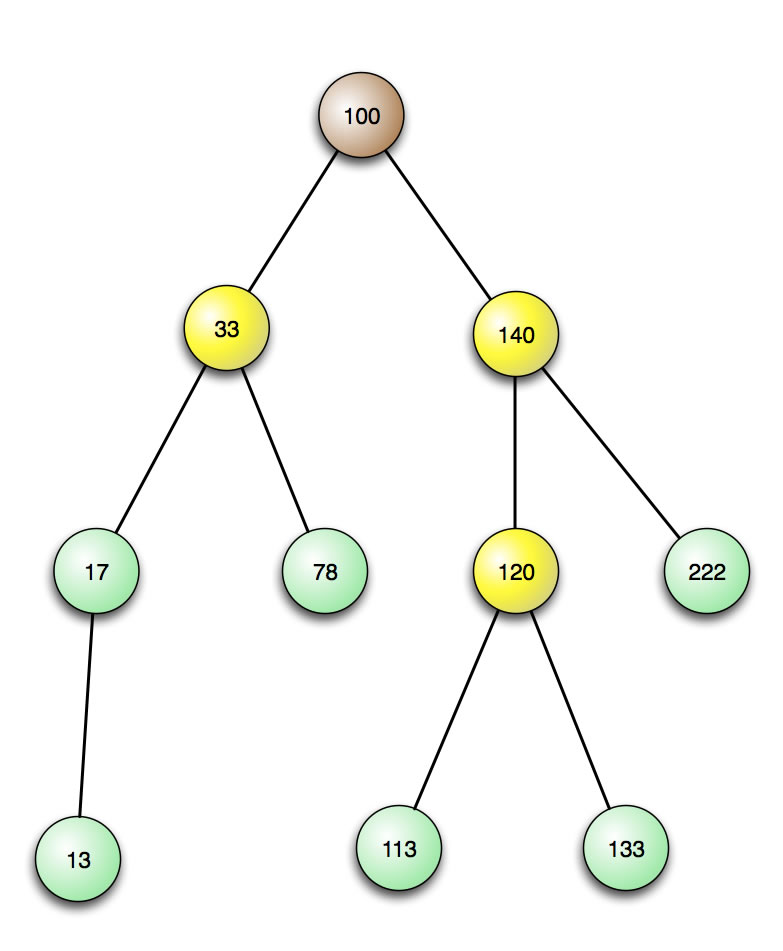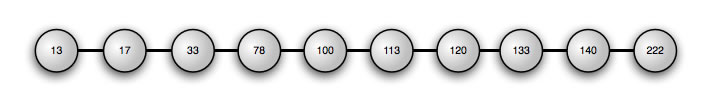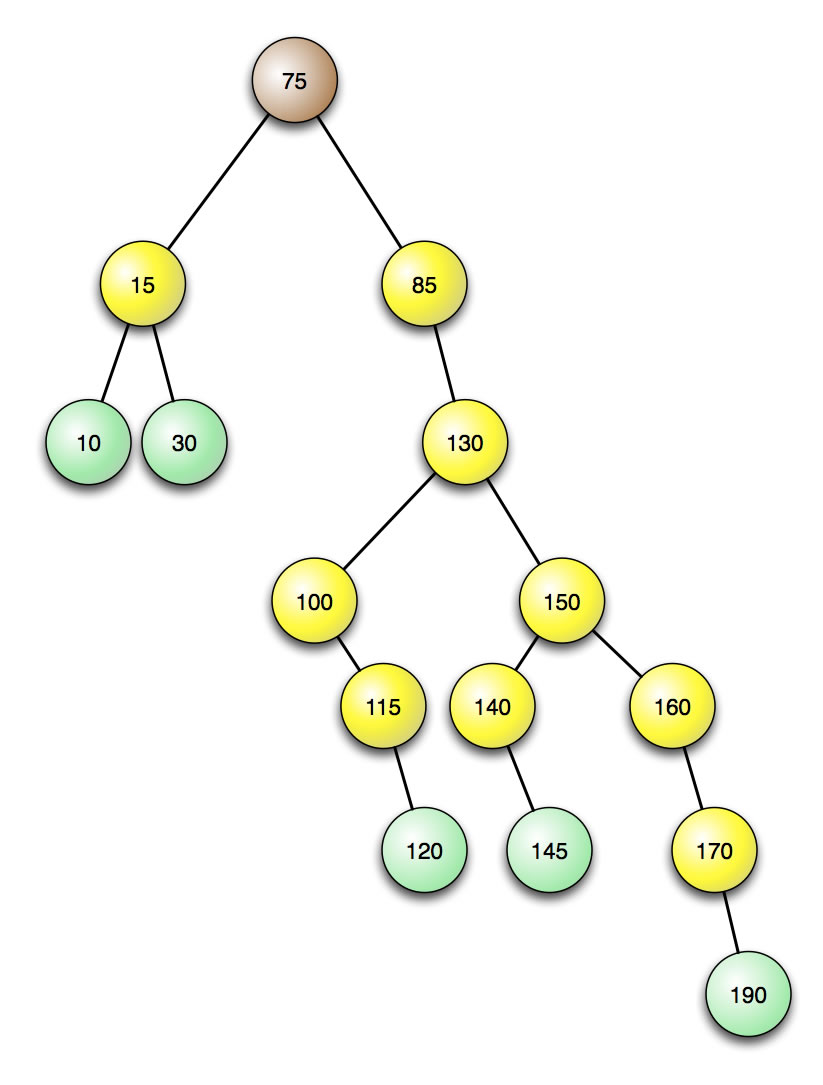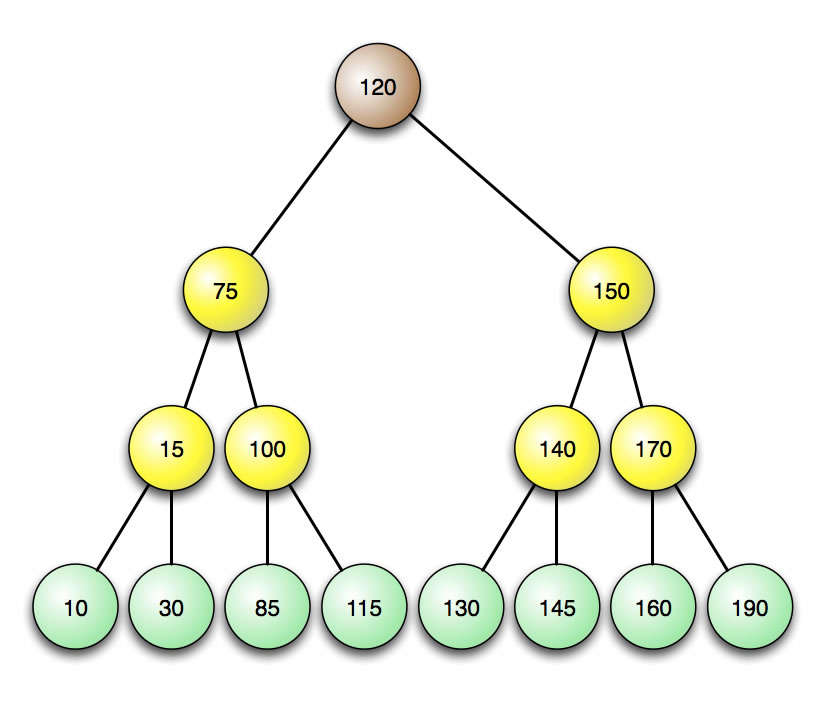# What is a binary search tree 1

## Binary search trees

### Basics

In theory, a tree can be completely disordered, even chaotic. As long as each node has at least two children, it is a tree. The definition of the term "tree" does not state how these successors have to be arranged or that they have to be ordered at all.

Such disordered trees are usually useless in computer science. Trees are mostly used to store data, and you want to find data as quickly as possible. Hence a tree has to be ordered. This can be achieved with the help of a certain class of trees, the so-called binary search trees.

The following figure shows such a binary search tree:A binary search tree

The content of the root contains the number 100. All nodes to the left of the root have smaller numbers as content, while all nodes to the right of the root have numbers that Not are smaller. This rule also applies to each of the inner nodes. For example, let's look at node 33. The successor on the left has a smaller value (17), the successor on the right has a larger value (78).

After this preliminary consideration, the definition of the term "binary search tree" should actually no longer be a problem:

##### binary search tree

A binary search tree is a binary tree in which the following applies to all subtrees:

1. the left subtree of a node only contains elements whose value is less than the value of the node.
2. the right subtree of a node only contains elements whose value is not smaller than the value of the node.
##### Binary search trees as ADTs

Incidentally, a binary tree is an abstract data type (ADT) because it can be defined simply by specifying its operations. Here is a possible example for a definition of the ADT "binary search tree":

The ADT binary tree

The abstract data type binary search tree is defined by the following operations:

• Init (): Creates an empty search tree.
• Insert (x): The element x is added to the left subtree if it is smaller than the root, otherwise to the right subtree.
• Remove (x): The element x is removed if it is present.
• Member (x): If the element x is present in the tree, TRUE is returned, otherwise FALSE.
• Empty (): If the binary tree is empty, TRUE is returned, otherwise FALSE.

Of course, you can include other operations in this definition, for example you could design operations that return the left or right subtree of the root as a value, and so on

### Advantage of binary search trees

What are the advantages of such a binary search tree compared to a doubly linked sorted list of elements? Let's take a look at a specific list. The pointers are not shown here for reasons of clarity.An ordered list of numbers

The element 78 is to be found by a linear search. Four comparisons are necessary for this, as you can easily see. The same search in our binary search tree from the figure above would only cost three comparisons. In order to find element 100 in the list, five comparisons would be necessary in a linear search. In the search tree we find the 100 after a comparison, because the 100 is the root of the tree.

You can see immediately that searching in the binary tree is much faster on average than in a sorted list.

##### For experts

Here one could of course object: If one were to search through the sorted list with a binary method, it would be just as fast as searching through a binary tree.

This objection is justified, but binary search is also a method in which a sorted list is temporarily transformed into a binary search tree. So you make use of the same technology that is in a binary search tree.

Now let's look at the following binary tree:A very right-biased binary tree

The 15 numbers are arranged in order; all numbers in the left subtree are smaller than the number in the root, and the non-smaller numbers are in the right subtree. Each node has a maximum of two successors, which means that all conditions for a binary search tree are met. However, this tree is not optimally structured. For example, to find the number 190 you need 7 comparisons, because the 190 is in the 7th level of the tree. In contrast, only 3 comparisons are required to find the 10. If you add everything up:

1x1 + 2x2 + 3x3 + 2x4 + 3x5 + 3x6 + 1x7 = 62

and divides the sum of the comparisons needed to find all the numbers by the number of numbers, i.e. 14, you get 62/15 = 4.13 comparisons on average. To find an (existing) number in this tree, an average of 4.13 comparisons are necessary.

The following binary search treeA fully balanced binary tree

contains the same 15 numbers. However, this binary search tree is optimally structured, it is completely balanced. A maximum of 4 comparisons are necessary to find a number. The exact calculation provides

1x1 + 2x2 + 4x3 + 8x4 = 49

To find a number here, 49/15 = 3.27 comparisons are necessary. That is a big step forward from the unbalanced tree, and a huge step up from an ordered linear list; this would require around 7.5 comparisons on average for 15 numbers.

##### For mathematicians:

The time behavior of the search in a binary search tree can be given by the formula

O (N) = log N

are characterized.

###### Reason:

A fully populated binary search tree with two levels has three nodes:

20 + 21 = 3

A fully populated binary search tree with three levels has seven nodes:

20 + 21 + 22= 7

To find the seven numbers in the fully occupied tree with three levels, a maximum of 3 comparisons are necessary. Now is a lie2(8) = 3 or log2(7) <3. And for the three numbers in levels 1 and 2, even fewer comparisons are necessary. So the claim O (N) = log N is absolutely true.